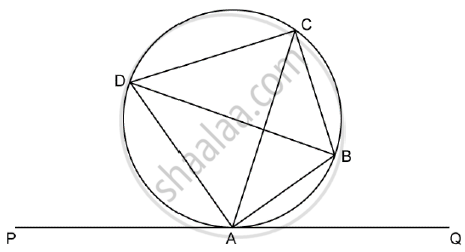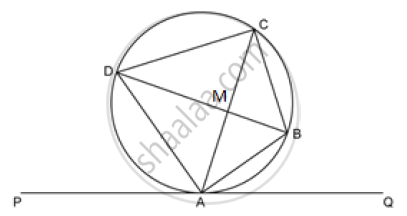Share

# In the Given Figure Pq is a Tangent to the Circle at A, Ab and Ad Are Bisectors of Anglecaq and Anglepac. If Anglebaq = 30^@. Prove That:Bd is a Diameter of the Circle and Abc is an Isosceles Triangle - ICSE Class 10 - Mathematics

ConceptConstruction of Tangents to a Circle

#### Question

In the given figure PQ is a tangent to the circle at A, AB and AD are bisectors of angleCAQ and angle PAC. if angleBAQ = 30^@. prove that:

1) BD is a diameter of the circle

2) ABC is an isosceles triangle#### Solution1) angleBAQ = 30^@

SinceAB is the bisector of angleCAQ

=> angleCAB = angleBAQ = 30^@

AD is the bisector of angle CAP and P-A-Q,

angle DAP + angle CAD + angle CAQ = 180^@

=> angleCAD + anglle CAD + 60^@ = 180 ^@

=> angle CAD = 60^@

So angle CAD + angle CAB = 60^@ + 30^@ = 90^@

Since angle in a semi-circle = 90°

⇒ Angle made by diameter to any point on the circle is 90°

So, BD is the diameter of the circle.

2) SinceBD is the diameter of the circle, so it will pass through the centre.

By Alternate segment theorem

angle ABD = angle DAC = 60^@

So, in angle BMA,

angle AMB = 90^@    .........(UseAngleSumProperty)

We know that perpendicular drawn from the centre to a chord of a circle bisects the chord.

=> angle BMA = angle BMC = 90^@

In triangleBMA and triangleBMC

angleBMA = angleBMC = 90^@

BM = BM (common side)

AM = CM(perpendicular drawn from the centre to a chord of a circle bisects the chord.)

⇒ ΔBMA ≅ ΔBMC

⇒ AB = BC (SAS congruence criterion)

⇒ ΔABC is an isosceles triangle.

Is there an error in this question or solution?

#### APPEARS IN

Solution In the Given Figure Pq is a Tangent to the Circle at A, Ab and Ad Are Bisectors of Anglecaq and Anglepac. If `Anglebaq = 30^@. Prove That:Bd is a Diameter of the Circle and Abc is an Isosceles Triangle Concept: Construction of Tangents to a Circle.
S